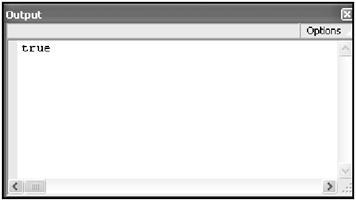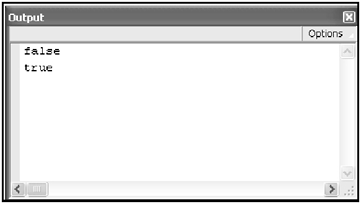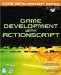# The Operators

 [ LiB ]

In this section, I am going to go over ActionScript's operators in detail. Operators are special symbols that modify and operate the contents of variablessome of these operations include addition, subtraction, multiplication, and division. There are a lot of these guys floating around, and every one of them is just as useful as the next . These operators can do quite a few things for youthey can do arithmetic, assign values, and if that wasn't enough, they can also compare values. Pay close attention to the assignment operator. Try not to confuse it with the comparison operator. I find that many novice programmers usually make the mistake of using one for the other.

#### Assignment Operators

We already met our friend the assignment operator. Let's see another example using the assignment operator:

` var anotherVar = 50.5; `

Here we see a new data type being assigned from the left. It's another number, you might sayalbeit a special type of numbercalled a floating point number. If that decimal point weren't there, the number would be considered an integer. You saw examples of those integers earlier. As for this example, anotherVar now has the value of 50.5. Notice that I avoided saying, "anotherVar is equal to 50.5." Even though this is true, the statement is not an equation. You will understand the difference when we go over the incrementing operators.

NOTE

NOTE

Just to review: you have already learned three kinds of data types.These are the string, the integer and the floating point type. I believe the use of data types cannot be learned in one section of a chapterthey must be used throughout the book in order to really get the hang of them.You will learn more compli cated data types as you advance through the chapters.

#### The Comparison Operators

Among the operators there are a few operators that help you compare values; these will be heavily used later in the chapter (and in the book) and are called comparison operators . These operators are ==, <, >, <=, >= and != .

##### The == Operator

I'm going to speak about the equality comparison operator first. That's == . Before I show you an example, allow me to introduce another data type. It is called the Boolean data type. This data type is only one of two values that can be represented by the keywords true and or false . A Boolean data type can also accept any non-zero number as a true value and zero number as a false value. Now that you have an idea of what a Boolean value is, allow me to finish defining the function of the comparison operator. The comparison is written as two assignment operators butted up against each other. Let's see it in action in the following listing.

` var temp1 = 5; var temp2 = 10; trace(temp1 == temp2); `

So what is happening here? The program creates two variables . One has the value of 5 and the other has the value of 10. The trace command is being treated differently yet again. It is set up to output the results from the comparisonbetween its parentheses.

You already know that this statement is false5 is not equal to ten. So what is the output? It's "false." If you were to type this listing in, save the project, and then run it, you will find the word false in the Output Window.

NOTE

CAUTION

Remember the difference between = and == . One assigns a value to a vari able and the other compares two values and/or variables to each other. It is a huge difference that can cause many hidden bugs within your program. If you find me repeating this caution again, it's because it's for your own good.

You might not find this operator immediately useful now, but just wait until I get to conditional statements.

For the sake of clarity, let's produce a true condition by modifying the previous listing.

` var temp1 = 7; var temp2 = 7; trace(temp1 == temp2); `

Type this listing in and test it for yourself. Once you verify this script, you should get the output shown in Figure 3.16.

##### Figure 3.16. The verified outputOkay, great. So now you know how to use the equality comparison operator. But I mentioned others, didn't I?

##### The != Operator

Introducing the not-equal-to operator, != . This operator checks for the opposite of what the comparison operator checks for. It checks to see if the left and right values are not equal to each other.

Check out the next listing. See if you can guess the output before even touching the computer.

` var temp1 = 7; var temp2 = 7; var temp3 = 8; `

` trace(temp1 != temp2); trace(temp1 != temp3); `

NOTE

NOTE

It is common in the industry to refer to whatever is on the left side of an assignment operator as the L-value and whatever is on the right side as the R-value . I'll keep my text simple but this is good for you to know.

The program creates three variables: temp1, temp2 and temp3. temp1 and temp2 are both equal to 7. temp3 is equal to 8. I have included two trace commands to verify our operator's function.

Step into the program. What is the first trace command doing? Read it out loud. It asks, "Is temp1 not equal to temp2? "

Replace the variables in this sentence with their values. You'll see it makes more sense this waythe same way that your computer sees it. "Is 7 not equal to 7?" We know this is false because 7 is equal to 7. Thus, "false" should be the first trace output.

What is the second line saying? "Is temp1 not equal to temp3? " After you replace these with their values you get: "Is 7 not equal to 8?" You know that this statement is true because you know that 7 has never been equal to 8. Therefore, you know that the second output should be the Boolean value of "true".

Go ahead and type in the listing, save it, and run it as usual. You should get a window similar to that in Figure 3.17.

##### Figure 3.17. Your final outputYou're doing well if you understand everything up to this point. It's going to be smooth sailing through the rest of the operators.

##### The <, <=, > and >= Operators

The next set of operators I want to introduce are the less than (<) and the greater than (>) signs. These operators came back to haunt you from your math class in junior high. But they are here to help you, not harm you. Welcome them.

Start a new project and open up the Actions Panel. Type in the following listing:

` var temp1 = 5; var temp2 = 8; var temp3 = 5; trace(temp1 > temp2); trace(temp1 < temp2); trace(temp1 >= temp3); trace(temp1 <= temp3); `

Notice how I sneaked the greater than or equal to (>=) and the less than or equal to (<=) operators into the program also.

You are familiar with most of this listing because you have been dealing with similar programs in past listings. You should be familiar with creating local variables and initializing them with a numeric value.

After creating three dummy variables for the program, it goes on to compare temp1 and temp2 . Notice carefullyit compares them twice. The first time, it asks, "Is temp1 is greater than temp2 ?" We know that temp1 is 5 and temp2 is 8. This tells us that the answer to our question is false5 is not greater than 8.

The second comparison asks, "Is temp1 less than temp2? " You know that 5 is less than 8, so this would yield "true." We're halfway through the listinglet's finish assuming the output.

The third trace command uses our greater than or equal to (>=) operator. Reading it out loud, it states, "Is temp1 greater than or equal to temp3? " Rephrased: "Is 5 greater than or equal to 5?" Awkward at first encounter, but think of it logically and it makes sense. 5 is not greater than 5, but the question has another partit also asks if 5 is equal to 5this is the true part. This trace command then outputs a "true" value.

The final trace command is not too different from the last. It asks, "Is temp1 less than or equal to temp3 ?" In other words, "Is 5 less than or equal to 5?" This is true because, again, 5 is equal to 5.

So what is our collected output?

` false true true true `

#### The Arithmetic Operators

Here are a few operators that you might use fairly often. The common ones are the addition (+), subtraction (-), multiplication (x), and division (/) operators. As if you didn't have enough in your arsenal, there are also the incrementing and the decrementing operators. These look like double pluses (++) and double minuses (--) , respectively.

These operators are best taught in code, so allow me to throw another listing at you. Notice the commenting. Get used to it.

` // Game Development with ActionScript // By Lewis Moronta (c) 2003 // This program demonstrates the use // of valuable ActionScript operators. // Feel free to use and modify. var temp01 = 5; var temp02 = 3; var temp03; // Notice: No initial value // BEWARE: This is not an equation! // If temp's value is 5, then evaluate // the R-value of the assignment operator // first. 5 + 1 = 6. Now that we have 6 // on the right side, assign it to temp01. // Conclusion? temp01 will be added one // to itself after this line. temp01 = 6; temp01 = temp01 + 1; // This does something similar, but // it's a shorthand form. If temp02 // is now 3, it will be added one // after this line. temp02++; // Let's verify trace(temp01); trace(temp02); // Subtract one from temp01 and temp02 temp01 = temp01 - 1; temp02--; trace(temp01); trace(temp02); // You can also calculate variable values // and assign the final value to another variable. temp03 = temp01 * temp02; trace(temp03); // The other shorthand operators are // very useful as well. These are the // +=, -=, *= and the /= operators. // This operator adds the R-value to // current L-value and stores it in // the variable on the left. Seems // complicated to explain but it's // very simply written. temp03 += 5; // Since temp03 was 15 and 5 was // added in the last line, it should // now be equal to 20--let's verify! trace(temp03); // Can you guess what its value will // be after this series of operations? temp03 -= 10; temp03 *= 10; temp03 /= 2; // Answer should be 50. Why? // temp03 was 20 before the series // of operations. 10 was subtracted // making it equal to 10. It then // was multiplied by 10 so it was // then equal to 100. The line after // that divided it by 2-so we ended // up with 50. Let's verify this. trace(temp03); `

A program can suddenly look long. This shouldn't intimidate youa game would definitely be longer than this. I heavily commented this code because a lot of what is going on in there we haven't gone over. Allow me to clarify a lot of it. Take a look at the following line:

` temp01 = temp01 + 1; `

For many novice programmers, this line is extremely hard to swallow the first time around. Why? Because your schooling brainwashed you to believe that this statement is an equation and would therefore impossiblehow could one variable be equal to the same variable plus one?

Remember that this isn't an equation. It is an assignment operation. Remember order of operations in school? This falls into a simple case of evaluating the right side of the operator before the left side. R-value before L-value. If anything were inside parentheses, it would be evaluated first. Multiplication and division are evaluated first, and then addition and subtraction. You must pay close attention to the unary incrementing and decrementing operators. Their execution depends on which side of the variable they are on. I'll discuss that in more detail when we go over conditionals and loops .

As for our expression, the addition operation is the only thing happening, and since it's on the right side, it gets executed first. temp01 started out as 5. 1 is being added to 5the answer is 6. This value is then pushed into the variable on the left side. temp01 is now equal to 6. The trace command soon after that verifies this. Remember to verify everything!

temp02 is being modified in a similar way. This time, we are using the unary incrementing operator. It adds 1 to its value. The trace command soon after that also verifies its new value, which should be 4.

Soon after, you see the following statements:

` temp01 = temp01 - 1; temp02--; `

NOTE

TIP

The Help file has a nice table that you can use to learn the operator precedence for all operators in ActionScript. Open the Help file and do a quick search on Operator List. It's a very neat table breaking down the relationship between all the operators used in ActionScript. See how helpful Help can be?

They are very similar to the last additive operators, but there's one difference. 1 is being subtracted from both of these statements.

In the first statement, you'll notice that 1 is being subtracted from temp01' s current value. This difference is 5. 5 is then assigned to temp01 .

temp02 is being operated on by the decrementing operator. All this operator does is subtract 1 from the variable. Easy, eh?

The following statement

` temp03 = temp01 * temp02; `

demonstrates the ability to multiply the values of two different variables.

NOTE

NOTE

Notice how I keep stressing what seems to be obvious.The reasoning behind this madness is because when starting out, it will take you a few tries to get it right, unless you have experience with these types of expressionslike in a similar lan guage like C++.

The next four important statements, excluding the trace commands, are all similar they take the R-value and add it to the L-value and then they store that value in the variable sitting on the left side of the operator. Let's see four examples that I extracted from the listing:

` temp03 += 5; temp03 -= 10; temp03 *= 10; temp03 /= 2; `

Allow me to run through the example. temp03 is 15 before hitting that line. Since it's an addition operation, we can see that 5 is being added to 15. temp03 becomes 20. Simple enough, no? 10 is then subtracted in the next line because of the subtraction operation. temp03 then becomes 10. The following line then multiplies 10 to the current value, which is 10. This leaves temp03 at 100. The final line divides temp03 by 2 and its final answer is left at 50.

NOTE

NOTE

You can put together very complex formulas and expressions on one line. For the sake of clarity, I will break down expressions over a few lines just for educational purposes. Once you feel confident enough, go ahead and code as you wish. An example would be: Dist = Math.sqrt ((x*x)+(y*y)); . That's actually the distance formulasee how different it looks in ActionScript?

And the final output?

` 6 4 5 3 15 20 50 `

Just like you predicted ! I think you're getting good at this. Let's move on.

 [ LiB ]Game Development with ActionScript
ISBN: 1592001106
EAN: 2147483647
Year: 2004
Pages: 162
Authors: Lewis Moronta